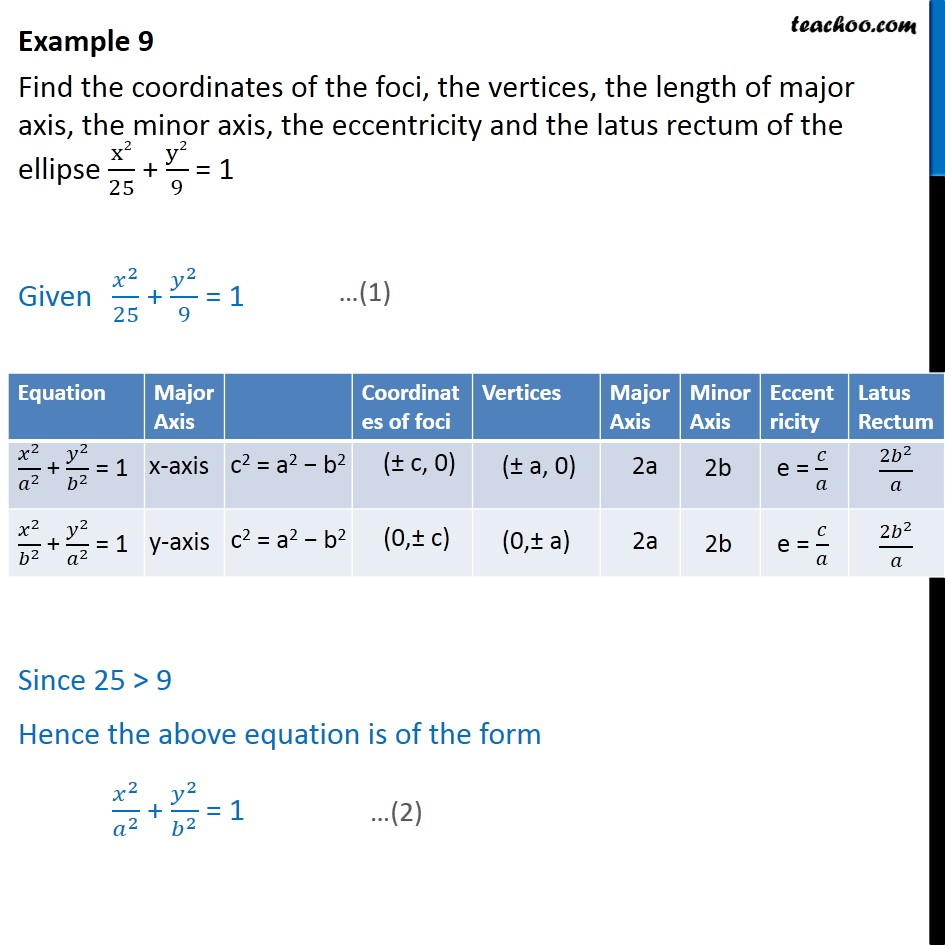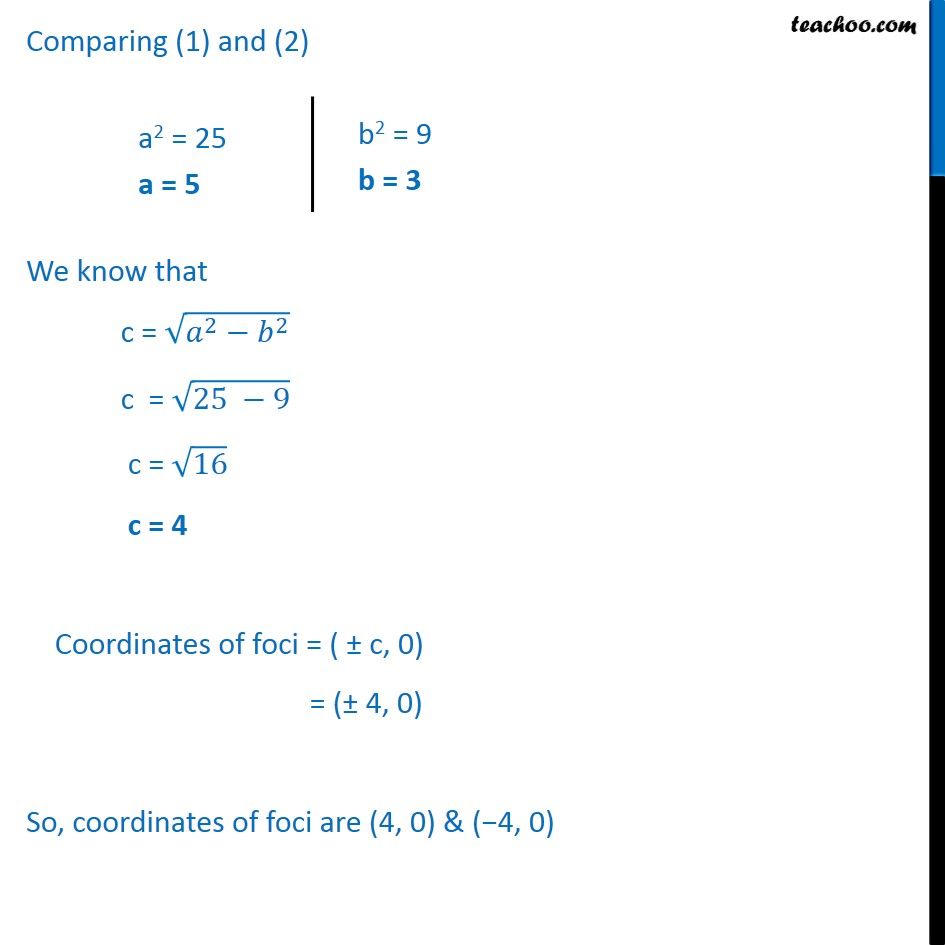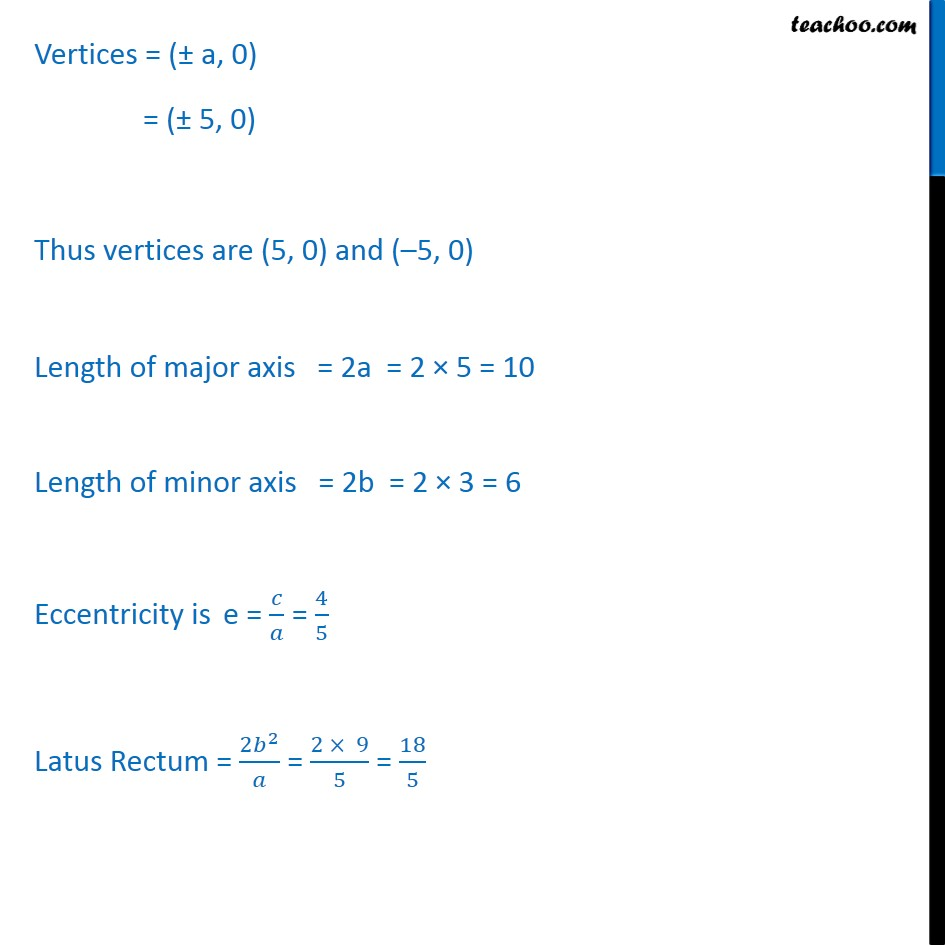1. Chapter 11 Class 11 Conic Sections
2. Serial order wise
3. Examples

Transcript

Example 9 Find the coordinates of the foci, the vertices, the length of major axis, the minor axis, the eccentricity and the latus rectum of the ellipse ﷐x2﷮25﷯ + ﷐y2﷮9﷯ = 1 Given ﷐﷐𝑥﷮2﷯﷮25﷯ + ﷐﷐𝑦﷮2﷯﷮9﷯ = 1 Since 25 > 9 Hence the above equation is of the form ﷐﷐𝑥﷮2﷯﷮﷐𝑎﷮2﷯﷯ + ﷐﷐𝑦﷮2﷯﷮﷐𝑏﷮2﷯﷯ = 1 Comparing (1) and (2) We know that c = ﷐﷮﷐𝑎﷮2﷯−﷐𝑏﷮2﷯﷯ c = ﷐﷮25 −9﷯ c = ﷐﷮16﷯ c = 4 Coordinates of foci = ( ± c, 0) = (± 4, 0) So, coordinates of foci are (4, 0) & (−4, 0) Vertices = (± a, 0) = (± 5, 0) Thus vertices are (5, 0) and (–5, 0) Length of major axis = 2a = 2 × 5 = 10 Length of minor axis = 2b = 2 × 3 = 6 Eccentricity is e = ﷐𝑐﷮𝑎﷯ = ﷐4﷮5﷯ Latus Rectum = ﷐2﷐𝑏﷮2﷯﷮𝑎﷯ = ﷐2 × 9﷮5﷯ = ﷐18﷮5﷯

Examples